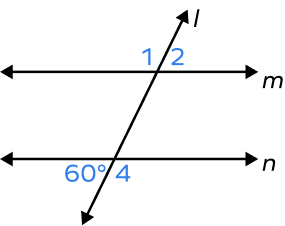# Alternate Exterior Angles – Definition, Theorem, Examples, FAQs

Home » Math-Vocabluary » Alternate Exterior Angles – Definition, Theorem, Examples, FAQs

## What Are Alternate Exterior Angles?

When two lines (parallel lines) are cut by a transversal at two distinct lines, a pair of angles that are created on the alternate sides of a transversal and on the outer side (exterior side) of the two lines are called alternate exterior angles.

What do alternate exterior angles look like?

The diagram on the left side shows the alternate exterior angles formed by two non-parallel lines cut by transversal. The diagram on the right shows the alternate exterior angles formed by two parallel lines cut by transversal.

## Alternate Exterior Angles: Definition

Definition of alternate exterior angles: A pair of angles formed on the alternate sides (opposite sides) of a transversal and on the exterior side of two lines cut by transversal are known as alternate exterior angles.

## Alternate Exterior Angles Theorem

The alternate exterior angle theorem states that when two parallel lines are cut by a transversal, then the pair of alternate exterior angles formed are congruent.

## Proof of Alternate Exterior Angles Theorem

Given: l || m and t is a transversal.

Alt tag: Parallel lines l and m cut by a transversal

To prove:

m∠1 = m∠8

m∠2 = m∠7

Proof:

Hence, proved.

## Converse of Alternate Exterior Angles Theorem

The converse of alternate exterior angles theorem states that if the alternate exterior angles formed by two lines cut by a transversal are congruent, then the two lines are parallel to one another.

## Facts about Alternate Exterior Angles

• Alternate exterior angles theorem is used to prove that two lines are parallel to each other.
• If the lines are not parallel, the alternate exterior angles are not congruent and do not have any relationship.

## Conclusion

In this article, we learned about Alternate Exterior Angles. We discussed the definition, the alternate exterior angles theorem, converse, and the proof. Let’s solve a few examples and practice problems for better comprehension.

## Solved  Examples on Alternate Exterior Angles

Example 1: Find the value of x.

Solution:

m∠EFH = 130o

m∠ACB = x

Here, m∠EFH + m∠GFH = 180o …angles in a linear pair

Thus, 130 + m∠GFH = 180o

m∠GFH = 180o – 130o

m∠GFH = 50o

Now, m∠GFH = x …Alternate Exterior Angles Theorem

Thus, x = 50o

Example 2: If two alternate exterior angles formed by two lines and a transversal are (2x + 10) and (4x – 20), then what should the value of x be such that two lines are parallel?

Solution:

For two lines to be parallel, the alternate exterior angles should be equal to each other.

2x + 10 = 4x – 20

4x – 2x = 20 + 10

2x = 30

$x = \frac{30}{2}$

x = 15

Thus, the two lines will be parallel for x = 15.

Example 3: Find the value of y if the two parallel lines are cut by a transversal.

Solution:

The given angles (3y + 49) and (7y – 55) are alternate exterior angles.

By the alternate exterior angles theorem, we have

(3y + 49) = (7y – 55)

4y = 104

y=26

## Practice Problems on Alternate Exterior Angles

1

### Alternate exterior angles formed by two parallel lines cut by a transversal are

Congruent
Non-congruent
complementary
supplementary
CorrectIncorrect
Alternate exterior angles are congruent when the two lines cut by a transversal are parallel.
2

### Find m∠2.$120^{\circ}$
$30^{\circ}$
$60^{\circ}$
$15^{\circ}$
CorrectIncorrect
Correct answer is: $60^{\circ}$
Alternate exterior angles formed by two parallel lines cut by a transversal are congruent.
The $60^{\circ}$ angle and ∠2 are alternate exterior angles formed by two parallel lines m and n cut by a transversal l are congruent.
Thus, m∠2 $= 60^{\circ}$
3

### Alternate exterior angles lie on the ______of the transversal.

opposite sides
Same side
outer sides
inner side
CorrectIncorrect
Alternate exterior angles lie on the opposite sides of the transversal.
4

opposite side
same side
interior region
outer side
CorrectIncorrect